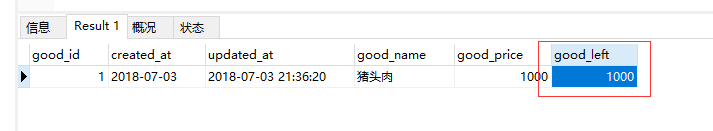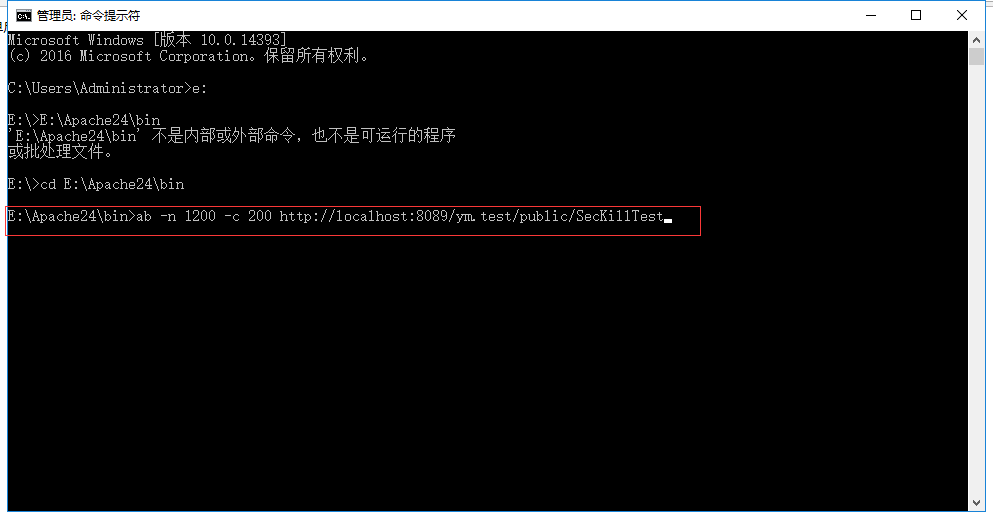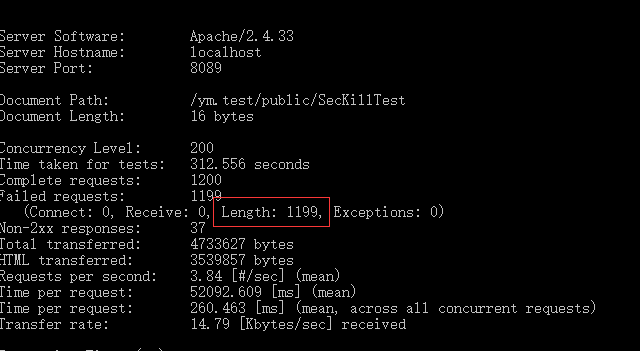laravel+Redis简单实现队列通过压力测试的高并发处理

2018/07/06 00:24

代码的流程

1.模拟用户请求，将用户写入redis队列中

2.从用户中取出一个请求信息进行处理（可以在这个步骤做更多的处理，请求过滤，订单复购等）

3.用户下单（支付等），减少库存。下面使用了两种方式进行了处理，一种使用了Redis中单线程原子操作的特性使程序一直线性操作从而保持了数据的一致

<?php

namespace App\Http\Controllers\SecKill;

use App\Http\Controllers\Controller;

class SecKillControllers extends Controller {

public function SecKillTest() {
///在此之前我们已经将一千过用户写入了redis中了
$num = Redis::lpop('user_list'); ///取出一个用户 /// ///一些对请求的处理 /// if (!is_null($num)) {
///将需要秒杀的商品放入队列中
$this->AddGoodToRedis(1); ///需要注意的是我们如果写的是秒杀活动的话，需要做进一步的处理，例如设置商品队列的缓存等方式，这里就实现了 ///下订单减库存$this->GetGood(1,$num); } } public function DoLog($log) { file_put_contents("test.txt", $log . '\r\n', FILE_APPEND); } /** * 重点在于Redis中存储数据的单线程的原子性，！！！无论多少请求同时执行这个方法，依然是依次执行的！！！！！ * 这种方式性能较高，并且确保了对数据库的单一操作，但容错率极低，一旦出现未可预知的错误会导致数据混乱； */ public function GetGood($id,$user_id) {$good = \App\Goods::find($id); if (is_null($good)) { $this->DoLog("商品不存在"); return 'error'; } ///去除一个库存$num = Redis::lpop('good_list'); ///判断取出库存是否成功 if (!$num) {$this->DoLog("取出库存失败"); return 'error'; } else { ///创建订单 $order = new \App\Order();$order->good_id = $good->good_id;$order->user_id = $user_id;$order->save(); $ok = DB::table('Goods') ->where('good_id',$good->good_id) ->decrement('good_left', $num); if (!$ok) { $this->DoLog("库存减少失败"); return; } echo '下单成功'; } } public function AddUserToRedis() {$user_count = 1000; for ($i = 0;$i < $user_count;$i++) { try { Redis::lpush('user_list', rand(1, 10000)); } catch (Exception $e) { echo$e->getMessage(); } } $user_num = Redis::llen('user_list'); dd($user_num); } public function AddGoodToRedis($id) {$good = \App\Goods::find($id); if ($good == null) { $this->DoLog("商品不存在"); return; } ///获取当前redis中的库存。$left = Redis::llen('good_list'); ///获取到当前实际存在的库存，库存减去Redis中剩余的数量。 $count =$good->good_left - $left; // dd($good->good_left); ///将实际库存添加到Redis中 for ($i = 0;$i < $count;$i++) { Redis::lpush('good_list', 1); } echo Redis::llen('good_list'); } public function getGood4Mysql($id) { DB::beginTransaction(); ///开启事务对库存以及下单进行处理 try { ///创建订单$order = new \App\Order(); $order->good_id =$good->good_id; $order->user_id = rand(1, 1000);$order->save(); $good = DB::table("goods")->where(['goods_id' =>$id])->sharedLock()->first(); //对商品表进行加锁(悲观锁) if ($good->good_left) {$ok = DB::table('Goods') ->where('good_id', $good->good_id) ->decrement('good_left',$num); if ($ok) { // 提交事务 DB::commit(); echo'下单成功'; } else {$this->DoLog("库存减少失败"); } } else { $this->DoLog("库存剩余为空"); } DB::rollBack(); return 'error'; } catch (Exception$e) { // 出错回滚数据 DB::rollBack(); return 'error'; //执行其他操作 } } }

AB测试

https://www.jianshu.com/p/43d04d8baaf70
0 收藏

作者的其它热门文章0 评论
0 收藏
0